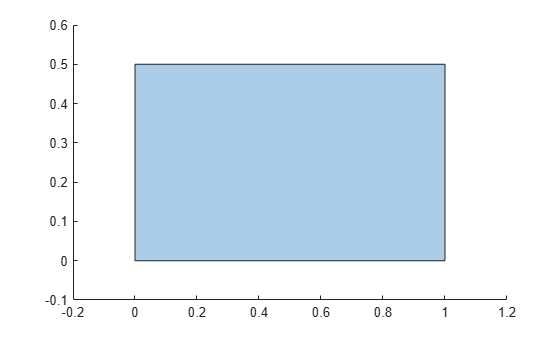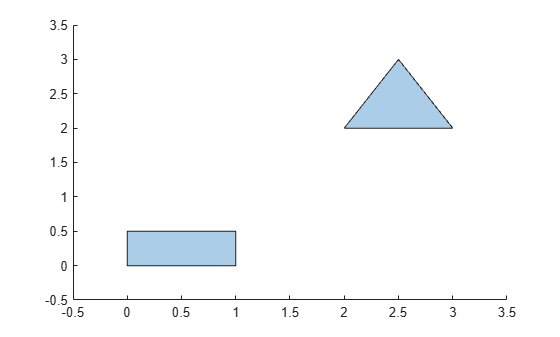Add `polyshape` boundary

## Syntax

``polyout = addboundary(polyin,x,y)``
``polyout = addboundary(polyin,P)``
``polyout = addboundary(polyin,{x1,x2,...,xM},{y1,y2,...,yM})``
``polyout = addboundary(___,Name,Value)``

## Description

example

````polyout = addboundary(polyin,x,y)` returns a `polyshape` object that is made up of an existing `polyshape` plus an additional boundary defined by the x-coordinates and the y-coordinates contained in the vectors `x` and `y`.```
````polyout = addboundary(polyin,P)` adds the boundary defined by the coordinates contained in the N-by-2 matrix `P`, where N is the number of vertices.```
````polyout = addboundary(polyin,{x1,x2,...,xM},{y1,y2,...,yM})` adds M boundaries, where the vectors of x-coordinates for each boundary are listed together in a cell array. The corresponding vectors of y-coordinates also are listed together in a cell array. Each xi must have the same length as the corresponding yi, but the number of vertices can vary among the boundaries.```
````polyout = addboundary(___,Name,Value)` specifies additional parameters for adding boundaries to a `polyshape` for any of the previous syntaxes.```

## Examples

collapse all

Create a rectangle, and then create a second polygon made up of the rectangle plus a triangle.

`polyin = polyshape([0 0 1 1],[0 0.5 0.5 0])`
```polyin = polyshape with properties: Vertices: [4x2 double] NumRegions: 1 NumHoles: 0 ```
`plot(polyin)``polyout = addboundary(polyin,[2 3 2.5],[2 2 3])`
```polyout = polyshape with properties: Vertices: [8x2 double] NumRegions: 2 NumHoles: 0 ```
`plot(polyout)`## Input Arguments

collapse all

Input `polyshape`, specified as a scalar.

Data Types: `polyshape`

x-coordinates of boundary vertices, specified as a vector. You can represent the coordinates of multiple boundaries simultaneously by placing a `NaN` between each boundary. For example, ```polyout = addboundary(polyin,[0 0 1 NaN 1 5 5],[1 0 0 NaN 5 5 1])``` returns a `polyshape` object made up of `polyin` plus two additional triangles.

Numeric input vertices that are not of type `double` are automatically converted to type `double`.

Data Types: `double` | `single` | `int8` | `int16` | `int32` | `int64` | `uint8` | `uint16` | `uint32` | `uint64`

y-coordinates of boundary vertices, specified as a vector. You can represent the coordinates of multiple boundaries simultaneously by placing a `NaN` between each boundary. For example, ```polyout = addboundary(polyin,[0 0 1 NaN 1 5 5],[1 0 0 NaN 5 5 1])``` returns a `polyshape` object made up of `polyin` plus two additional triangles.

Numeric input vertices that are not of type `double` are automatically converted to type `double`.

Data Types: `double` | `single` | `int8` | `int16` | `int32` | `int64` | `uint8` | `uint16` | `uint32` | `uint64`

Boundary vertices, specified as a 2-column matrix. The first column of `P` contains the x-coordinates of the vertices, and the second column contains the y-coordinates. `P` must have at least 3 rows.

You can represent the coordinates of multiple boundaries simultaneously by placing a `NaN` between each boundary. For example, ```polyout = addboundary(polyin,[1 0; 0 0; 0 1; NaN NaN; 1 5; 5 5; 5 1])``` returns a `polyshape` object made up of `polyin`, plus two additional triangles.

Numeric input vertices that are not of type `double` are automatically converted to type `double`.

Data Types: `double` | `single` | `int8` | `int16` | `int32` | `int64` | `uint8` | `uint16` | `uint32` | `uint64`

x-coordinates for M boundaries, specified as a cell array of vectors. The length of each xi can vary, but must match the length of the corresponding yi vector.

Numeric input vertices that are not of type `double` are automatically converted to type `double`.

Data Types: `cell`

y-coordinates of M boundaries, specified as a cell array of vectors. The length of each yi can vary, but must match the length of the corresponding xi vector.

Numeric input vertices that are not of type `double` are automatically converted to type `double`.

Data Types: `double` | `single` | `int8` | `int16` | `int32` | `int64` | `uint8` | `uint16` | `uint32` | `uint64`

### Name-Value Arguments

Example: ```polyout = addboundary(polyin,x,y,'SolidBoundaryOrientation','ccw')```

Specify optional pairs of arguments as `Name1=Value1,...,NameN=ValueN`, where `Name` is the argument name and `Value` is the corresponding value. Name-value arguments must appear after other arguments, but the order of the pairs does not matter.

Before R2021a, use commas to separate each name and value, and enclose `Name` in quotes.

Boundary orientation, specified as the comma-separated pair consisting of `'SolidBoundaryOrientation'` and one of these values:

• `'auto'` — Automatically choose vertex orientation as clockwise or counterclockwise for solid boundaries.

• `'cw'` — Treat clockwise vertex orientation as a solid boundary.

• `'ccw'` — Treat counterclockwise vertex orientation as a solid boundary.

The vertex orientation is not important for most applications and is primarily an algorithmic tool for determining boundary nesting. This parameter is typically specified for consistency and efficiency purposes when polygon data is produced by other software using a particular convention.

Vertex alteration, specified as the comma-separated pair consisting of `'Simplify'` and one of these values:

• `true` — Alter polygon vertices to produce a well-defined polygon when the input vertices produce intersections or improper nesting.

• `false` — Do not alter input vertices regardless of intersections or improper nesting. Computing with ill-defined polygons can lead to inaccurate or unexpected results.

Data Types: `logical`

Collinear vertices, specified as the comma-separated pair consisting of `'KeepCollinearPoints'` and one of the following:

• `false` — Remove collinear points so that the output `polyshape` contains the fewest vertices necessary to define the boundaries.

• `true` — Keep all collinear points as vertices.

When `'KeepCollinearPoints'` is not specified, its value is automatically set to the value used when creating the input `polyshape`.

Data Types: `logical`

## Version History

Introduced in R2017b